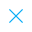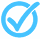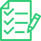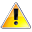Call Support +91-85588-96644

Keep me logged in
You can't leave Captcha Code empty
By submitting this form, you agree to the Terms & Privacy Policy.
ORTests given

OR# Probability VBQ (Class - X) Test - 1

Questions

Time

Highest
score

Level

## ENGLISH

Language

Topics Covered:Calculating Probability in Simple Independent EventsCalculating Probability in Simple Independent EventsCalculating Probability in Simple Independent EventsCalculating Probability in Simple Independent EventsCalculating Probability in Simple Independent EventsCalculating Probability in Simple Independent EventsCalculating Probability in Simple Independent EventsCalculating Probability in Simple Independent EventsCalculating Probability in Simple Independent EventsCalculating Probability in Simple Independent Events

### Latest Tests#### Mathematics Standard FLT - 1 (Class - 10)

00 Min - 40 Ques

Take Test#### Probability - III (Class X)

20 Min - 15 Ques

Take Test#### Surface Area and Volume (Set - I)

20 Min - 12 Ques

Take TestThis is a concept-building practice test and may not have exact structure as you would expect in the actual exam. Please exercise your discretion to attempt it or go to structured Featured Section.

Description:
Probability VBQ (Class - X) Test - 1